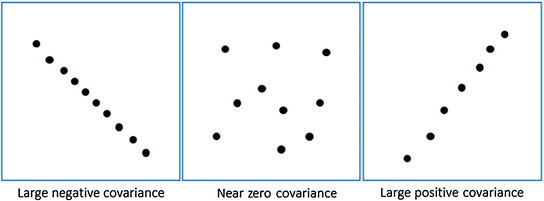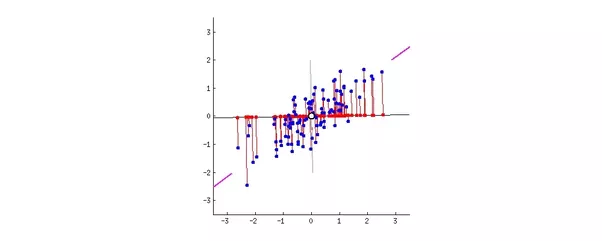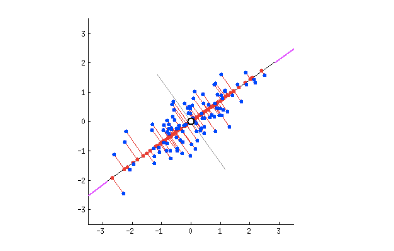# 目录

• 本文翻译自 Rishav Kumar 于 2019 年 1 月 2 日发表的文章 Understanding Principal Component Analysis
• 文中括号或者引用块中的 斜体字 为对应的英文原文或者我自己注释的话（会标明「译者注」），否则为原文中本来就有的话
• 文中的「我」均指原作者 Rishav Kumar
• 目录保留英文原文
• 本人水平有限，如有错误欢迎指出
• 能力尚可建议阅读英文原文

• 较少冗余的维度
• 仅仅保留最重要的维度

break1Continue break1

PCA 试图寻找一组新的维度（或者叫一组基础视图），使得所有维度都是正交的（所以线性无关），并根据数据在他们上面的方差进行排序。这就意味着越重要的成分越会排在前面（越重要 = 更大方差/数据分布更广）

PCA 的步骤如下：

1. 计算数据点的协方差矩阵 $X$
2. 计算特征向量和相应的特征值
3. 根据特征值，降序排列对应的特征向量
4. 选择前 $k$ 个特征向量作为新的 $k$ 维
5. 将原始的 $n$ 维数据变换为 $k$ 维

• 每个维度上的方差作为主对角线元素
• 每两个维度之间的协方差作为非对角线元素

• 主对角线元素的值比较大
• 非对角线元素为 0

• 找到可以无损地表示原始数据的线性无关的维度（或者基础视图）
• 这些新找到的维度应该能够让我们预测或者重建原始维度，同时应该最小化重建或者投影误差• 投影误差比第一条直线小
• 与第一种情况相比，投影后的数据点分布更广，即方差更大

• 我们已经计算了原始数据矩阵 $X$ 的协方差矩阵

$X:m \times n$

$P:k \times m$

$Y=PX:(k \times m)(m \times n)=(k \times n)$

• 定理 1：正交矩阵的逆是其转置，为什么？

令 $A$ 是一个 $m \times n$ 的正交矩阵（译者注：根据定义，正交矩阵一定是方阵，此处应 $m=n$），$a_i$ 是第 $i$ 个列向量，矩阵 $A^TA$ 的第 $ij$ 个元素为：

即 $A^TA = I$，所以 $A^{-1} = A^T$

• 定理 2：

令 $A$ 是一个实对称矩阵，$\lambda_1,\lambda_2,\cdots,\lambda_k$ 是 $A$ 的不同的特征值，$u_i \in R^n$ 非零，且 $1 \leq i \leq k$，那么 ${u_1,u_2,\cdots,u_k}$ 就组成一个正交规范集（orthonormal set）。

证明：

对于 $i \neq j$，且 $1 \leq i,j \leq k$，由于 $A^T = A$，我们有

由于 $i \neq j$，我们有 $\lambda_i \neq \lambda_j$，所以 $\langle u_i, u_j \rangle = 0$。

• 定理 3：

令 $A$ 为 $n \times n$ 的实对称矩阵且所有特征值都不相同，那么存在一个正交矩阵 $P$，使得 $P^{-1}AP=D$，其中 $D$ 为对角阵，对角元素为 $A$ 的特征值。

证明：

令 $A$ 有特征值 $\lambda_1,\lambda_2,\cdots,\lambda_n$，$u_i \in R^n$ 且 $|u_i| = 1$，$Au_i=\lambda_iu_i$，$1 \leq i \leq n$。通过推论，矩阵 $P=[u_1,u_2,\cdots,u_n]$ 是可逆的且 $P^{-1}AP=D$，是一个对角阵。而且，由定理 2，$(u_1,u_2,\cdots,u_n)$ 是一个正交规范集，所以 $P$ 确实是一个正交矩阵。

vectors
）。

vectors 的区别可参见 6.3 Orthogonal and orthonormal vectors - UCL，我摘抄部分如下：

Definition. We say that 2 vectors are orthogonal if they are perpendicular to each other. i.e. the dot product of the two vectors is zero.

Definition. A set of vectors S is orthonormal if every vector in S has magnitude 1 and the set of vectors are mutually orthogonal.

• X 的主成分是 $C_x$ 的特征向量
• $C_y$ 的第 $i$ 个对角元素是 $X$ 在 $i$ 维度上的方差

Note：PCA 是一种分析方法。你可以使用 SVD 做 PCA，或者使用特征分解（就像我们这里做的一样）做 PCA，或者使用其他方法做 PCA。SVD 只是另一种数值方法。所以不要混淆 PCA 和 SVD 这两个术语。但是有时我们会因为性能因素选择 SVD 而不是特征分解或者其他方法（这个不用关心），我们会在接下来的文章中探索 SVD。